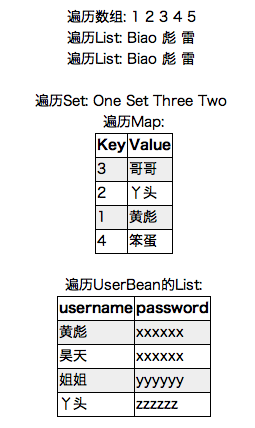## Java：JSTL遍历数组，List，Set，Map等_qinshang007的博客-程序员秘密<%@ page language="java" contentType="text/html; charset=UTF-8"

pageEncoding="UTF-8"%>

<%@ taglib prefix="c" uri="http://java.sun.com/jsp/jstl/core"%>

<%@ page import="java.util.*"%>

<%@ page import="bean.User"%>

<!DOCTYPE html PUBLIC "-//W3C//DTD HTML 4.01 Transitional//EN" "http://www.w3.org/TR/html4/loose.dtd">

<%

int[] ages = { 1, 2, 3, 4, 5 }; // 普通数组，JSTL直接使用JSP赋值表达式来取

List<String> names = new LinkedList<String>(); // List

request.setAttribute("names", names); // 添加到request

Set<String> set = new TreeSet<String>(); // Set

Map<String, String> map = new HashMap<String, String>(); // Map

map.put("1""黄彪");

map.put("2""丫头");

map.put("3""哥哥");

map.put("4""笨蛋");

List<User> users = new ArrayList<User>(); // JavaBean的List

session.setAttribute("users", users); // 添加到session

%>

<html>

<meta http-equiv="Content-Type" content="text/html; charset=UTF-8">

<title>Iterator Collections</title>

<style type="text/css">

table {

border-collapsecollapse;

border1px solid black;

}

td, th {

border1px solid black;

}

tr:nth-child(even) {

background#eee;

}

</style>

<body>

<center>

<c:forEach var="age" items="<%= ages %>">

<c:out value="\${age}" />

</c:forEach>

<br />

<c:forEach var="name" items="<%= names %>">

<c:out value="\${name}" />

</c:forEach>

<br />

<c:forEach var="name" items="\${names}">

<c:out value="\${name}" />

</c:forEach>

<br />

<br />

<c:forEach var="entry" items="<%= set %>">

<c:out value="\${entry}" />

</c:forEach>

<br />

<table>

<tr>

<th>Key</th>

<th>Value</th>

</tr>

<c:forEach var="entry" items="<%= map %>">

<tr>

<td><c:out value="\${entry.key}"/></td>

<td><c:out value="\${entry.value}"/></td>

</tr>

</c:forEach>

</table>

<br />

<table>

<tr>

</tr>

<c:forEach var="user" items="\${users}">

<tr>

</tr>

</c:forEach>

</table>

</center>

</body>

</html>

### C++自学第四天----枚举和typedef 声明_c++ enum typedef_爱做梦的鱼的博客-程序员秘密

1、枚举枚举类型(enumeration)是C++中的一种派生数据类型，它是由用户定义的若干枚举常量的集合。如果一个变量只有几种可能的值，可以定义为枚举(enumeration)类型。所谓"枚举"是指将变量的值一一列举出来，变量的值只能在列举出来的值的范围内。创建枚举，需要使用关键字 enum。枚举类型的一般形式为：enum 枚举名{标识符[=整型常数],标识符[=整型常数],…标...

1\